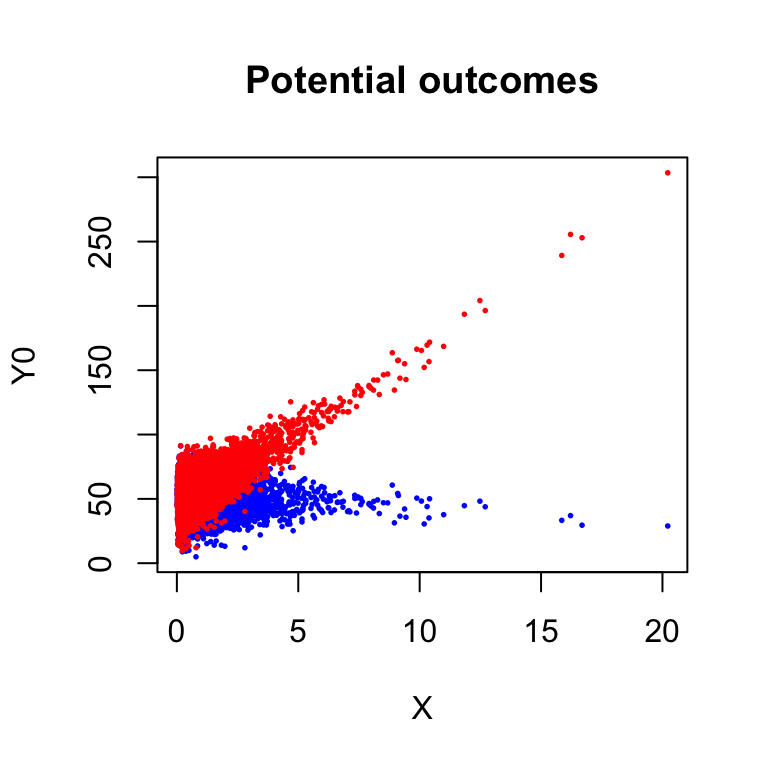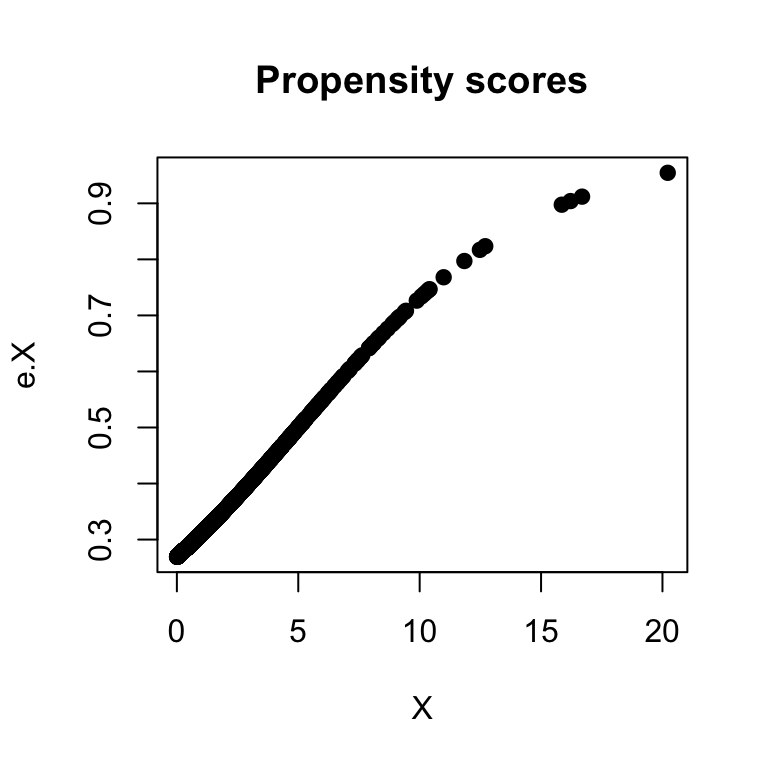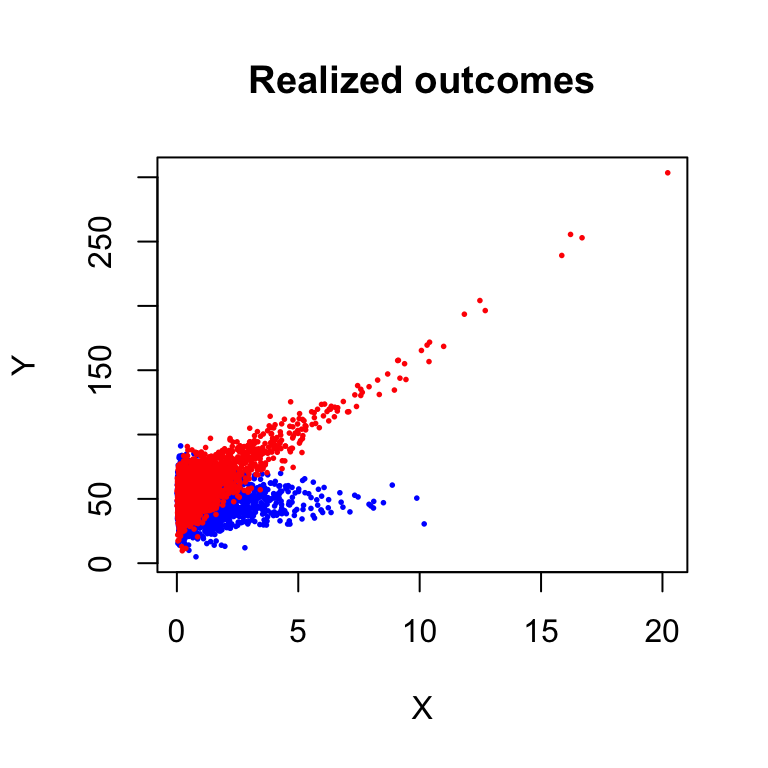## Inverse Propensity Score Weighting (IPW)

Recall that the stabilized IPW estimator for the ATE is $\hat{\rho}_{IPW} = \frac{\sum_{i=1}^n \frac{D_i}{\hat{e}(X_i)}Y_i}{\sum_{i=1}^n \frac{D_i}{\hat{e}(X_i)}} - \frac{\sum_{i=1}^n \frac{1-D_i}{1-\hat{e}(X_i)}Y_i}{\sum_{i=1}^n \frac{1-D_i}{1-\hat{e}(X_i)}},$ and that this can be implementing with weighted least squares regression in which you regress $$Y$$ on $$D$$, using the inverse of the estimate propensity score $$\hat{e}(X_i)$$ as the weights for the treated and the inverse of $$1-\hat{e}(X_i)$$ as the weights for the control.

Inference for $$\hat{\rho}_{IPW}$$ should account for the sampling variation in the estimated propensity scores, $$\hat{e}(\cdot)$$. In the Robust Inference I lecture notes, we showed how to derived the variance when $$\hat{e}(\cdot)$$ is estimated using an $$M$$-estimator. Here we will use the bootstrap instead.

### Make a simulated population with confounding

We will simulate a population with confounding in $$X$$. We do it in a way that makes it so that simple control specifications for $$X$$ in a regression would be inadequate, and so the specification challenge is a little harder than usual:

# Make population data
set.seed(11)
N.pop <- 10000
index <- 1:N.pop
X <- .5*exp(rnorm(N.pop))
Y0 <- 50-.05*X^2+(1+.5*(max(X)-X))*rnorm(N.pop)
Y1 <- -1.5 + Y0 + 10*X + .2*X^2+ .25*X*rnorm(N.pop)
e.X <- (1+exp(1-.2*X))^(-1)
D <- rbinom(N.pop, 1, e.X)
Y <- D*Y1 + (1-D)*Y0
rho <- mean(Y1-Y0)

plot(X, Y0, col="blue", pch=19,
cex=.25, ylim=range(c(Y1,Y0)),
main="Potential outcomes")
points(X, Y1, col="red", pch=19, cex=.25)plot(X, e.X, pch=19, main="Propensity scores")plot(X, Y, type="n", ,
main="Realized outcomes")
points(X[D==0], Y[D==0], col="blue", pch=19, cex=.25)
points(X[D==1], Y[D==1], col="red", pch=19, cex=.25)rho
##  7.124273
summary(lm(Y~D))[][,1]
## (Intercept)           D
##   49.800748    9.237881
summary(lm(Y~D+X))[][,1]
## (Intercept)           D           X
##   45.379997    7.790128    5.881640
summary(lm(Y~D*I(X-mean(X))))[][,1]
##      (Intercept)                D   I(X - mean(X)) D:I(X - mean(X))
##       49.7601797        7.3606239       -0.5394921       11.7581545
pop.data <- data.frame(index, Y, D, X)

### Sampling process

Next we draw a sample and estimate the effect using simple regression strategies and then IPW:

# Draw a sample:
n.samp <- 1000

samp.i <- sample(index, n.samp)
samp.data <- pop.data[samp.i,]
plot(samp.data$X, samp.data$Y, type="n")
points(samp.data$X[D==0], samp.data$Y[D==0], col="blue")
points(samp.data$X[D==1], samp.data$Y[D==1], col="red")